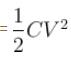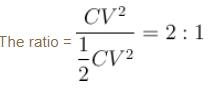Courses

# Work And Energy In Electrostatics System MCQ

## 10 Questions MCQ Test Topic wise Tests for IIT JAM Physics | Work And Energy In Electrostatics System MCQ

Description
This mock test of Work And Energy In Electrostatics System MCQ for Physics helps you for every Physics entrance exam. This contains 10 Multiple Choice Questions for Physics Work And Energy In Electrostatics System MCQ (mcq) to study with solutions a complete question bank. The solved questions answers in this Work And Energy In Electrostatics System MCQ quiz give you a good mix of easy questions and tough questions. Physics students definitely take this Work And Energy In Electrostatics System MCQ exercise for a better result in the exam. You can find other Work And Energy In Electrostatics System MCQ extra questions, long questions & short questions for Physics on EduRev as well by searching above.
QUESTION: 1

### When the separation between two charges is increased, the electric potential energy of the charges

Solution:

The correct answer is: may increase or decrease

QUESTION: 2

### Three particles, each having a charge of 10µC are placed at the corners of an equilateral triangle of side 10 cm. The electrostatic potential energy of the system is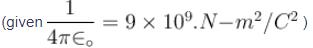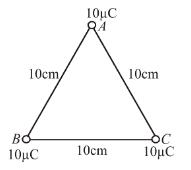Solution: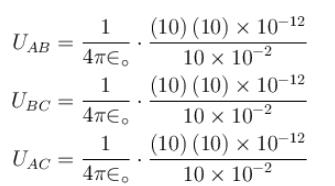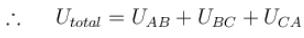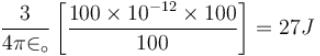The correct answer is: 27 J

QUESTION: 3

### If E is the electric field intensity of an electrostatic field then the electrostatic energy density is proportional to.

Solution: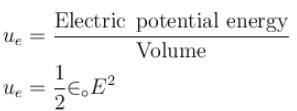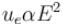QUESTION: 4

A point charge q is surrounded by six identical charges at distance r shown in figure. How much work is done by force of electrostatic repulsion, when the point charge at the centre is removed to infinity?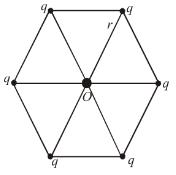Solution:

Work done in electrostatic system = change in electrostatic energy,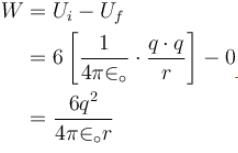The correct answer is: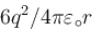QUESTION: 5

If a positive charge is shifted from a low-potential region to a high potential region. The electric potential energy.

Solution:

QUESTION: 6

How much kinetic energy will be gained by an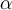particle in going from a point at 70 V to another point at 50 V?

Solution: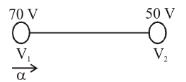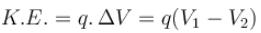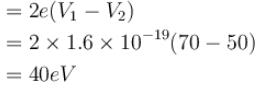The correct answer is: 40 eV

QUESTION: 7

A charge Q is uniformly distributed over the surface of a spherical shell of radius R. The electrostatic energy is directly proportional to :

Solution:

Electric field is given by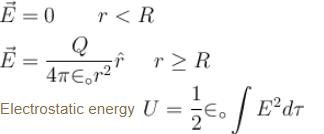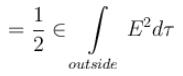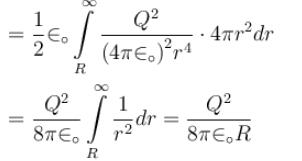The correct answer is: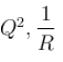QUESTION: 8

Two conducting sphere of radii R1 and R2 are charged with charges Q1 and Q2 respectively. On bringing them in contact there is.

Solution:

Potential at the surface of sphere of radius R1 is equal to V1 at the surface of sphere of radius R2 is equal to V2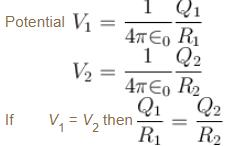or  Q1R2  =  Q2R1
In this case, there will be no flow of charges. However, If the given sphere have unequal potential then the charges shall flow from higher potential to lower potential. Flow of charges shall be accompanied by loss of energy.
The correct answer is: no increase in the energy of the system

QUESTION: 9

An ion with charge +q is in a region of uniform electric field of magnitude E. The charge is moved a distance d in the direction of field. Which of the following is true?

Solution:

Electric force,  F = qE
Work done = W = F.d = qEd.
But the change is electrostatic energy = –W
So potential energy decreased.

The correct answer is: The electric potential energy of the ion decreased by the amount qEd.

QUESTION: 10

A capacitor is charged to battery potential V in a RC circuit. The ratio of the energy supplied by the battery and that stored in the capacitor is :

Solution:

When a capacitor is connected to a battery. Then charge on the capacitor after a long time is q = CV
The work done by the battery or energy supplied by battery
= charge × voltage
CV × V = CV2
Now, electrostatic energy in capacitor